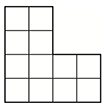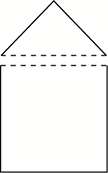Composition of Geometric Figures

My Definition

Key Characteristics

The following are true of composition of geometric figures:

• These terms refer to taking geometric figures apart or putting them together to form new figures.

• Sometimes, these acts lead to the creation of a new shape or shapes with different attributes.

• When composing with geometric figures, overlap does not occur.

Example

The composite figure below is composed of two rectangles.Click on the link below to further study examples of composition of geometric figures.

Composition of Geometric Figures Activity

Non-example

The following figures are overlapping, and as such are not a composition of figures.The pentagon below has been decomposed to create a square and a triangle.TEKS: 1(6)(F), 2(8)(D), 6(8)(B)